# Search

About 15 Search Results Matching Types of Worksheet, Worksheet Section, Generator, Generator Section, Subjects matching Math, Type matching Worksheet Section, Grades matching 4th Grade, Similar to Valentine's Day Picture Matching Worksheet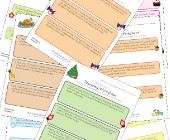## Rounding Worksheets

Check out our collection of rounding worksheets in...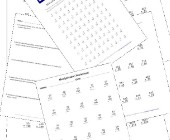## Multiplication Worksheets for Kids

This set of math worksheets is geared towards help...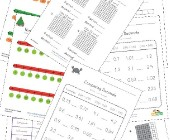## Decimal Worksheets

This collection of free decimal worksheets will he...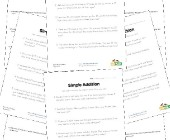## Word Problem Worksheets

Help children develop their problem solving skills...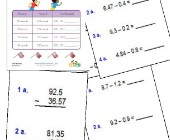## Subtracting Decimals Worksheets

These worksheets will help children practice decim...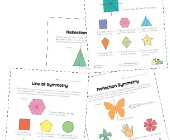## Symmetry Worksheets

Help kids learn about the concept of symmetry with...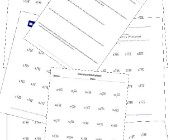## Division Worksheets

Help kids learn division with this collection of m...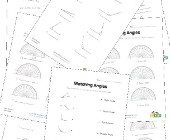## Angles Worksheets

Help kids learn about angles with this collection ...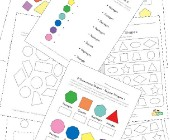## Geometry Worksheets

Help kids learn the early basics of geometry inclu...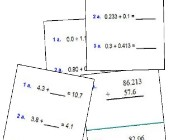Help kids practice decimal addition with the follo...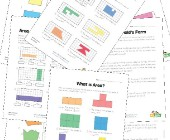## Area and Perimeter Worksheets

Help kids learn to calculate the area and perimete...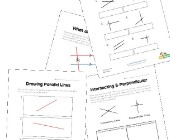## Parallel, Perpendicular and Intersecting Lines Worksheets

Help kids learn about lines including parallel lin...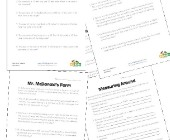## Area and Perimeter Word Problems

This collection of area and perimeter worksheets c...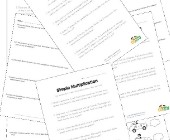## Multiplication Word Problems

Practice multiplication and problem solving with t...